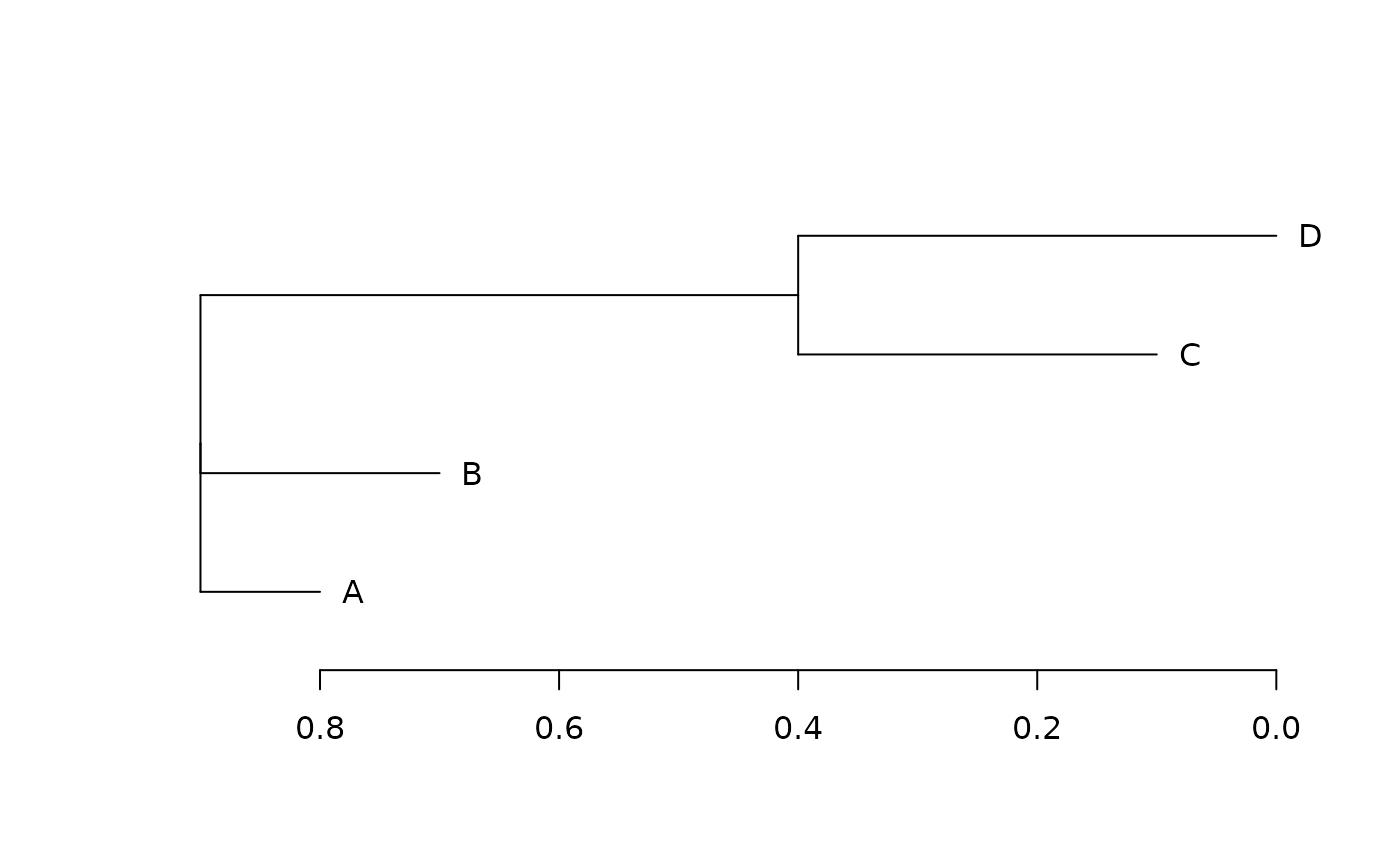`reposition` is a helper function used for manually creating `"dendrogram"` objects from nested lists. The function recursively reassigns the 'height' attributes at each node by a given constant.

## Usage

``reposition(x, shift = "reset")``

## Arguments

x

an object of class `"dendrogram"`.

shift

either the character string "reset" (shift the graph so that the height of the farthest leaf from the root is zero), or a numeric value giving the amount to shift the graph along the primary axis.

## Value

Returns an object of class `"dendrogram"`.

Shaun Wilkinson

## Examples

``````  x <- read.dendrogram(text = "(A:0.1,B:0.2,(C:0.3,D:0.4):0.5);")
plot(x, horiz = TRUE)
x <- reposition(x)
plot(x, horiz = TRUE)``````